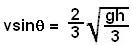## Saturday, May 3, 2008

### Irodov Problem 1.136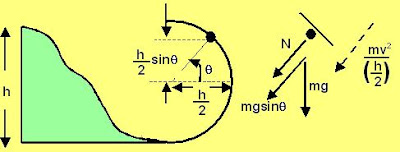As the mass slides down the slope it looses it potential energy and gains kinetic energy and so it velocity increases. At the base of the loop it has its maximum velocity. From this point its starts to climb up the loop and starts to loose its kinetic energy as it gains potential energy. When the particle is at an angle, as seen from the figure it will be at a height offrom the ground. Let the speed of the particle be v at this point. Then the net change in potential energy must be equal to the particle's kinetic energy. At this point the particle has lost a height of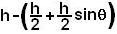and hence we have,At this point there are two forces acting on the particle, i) the normal reaction from the surface N
and ii) the force of gravity mg. Since the particle is rotating in a circular path with radius h/2 it also experiences a centripetal acceleration of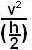. Hence along the direction perpendicular to the surface we have,At the point when the mass looses contact with the surface, the normal reaction becomes zero, i.e. N=0. Using this condition in (1) and (2) we have,At this point we can use (1) to compute the velocity of the particle as,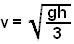AT this point however, the particles velocity is directed along the slope of the circular surface. The horizontal component of the particle is then given by